# Physics 3: Springs & Waves Flashcards Preview

## MCAT > Physics 3: Springs & Waves > Flashcards

Flashcards in Physics 3: Springs & Waves Deck (36):
1

2

3

## Oscillating Systems4

5

6

7

8

9

10

11

## Comparing: mass-spring system and the simple pendulum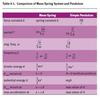12

13

## Transverse Waves

### sinusoidal waves a waveform in which the direction of particle oscillation is perpendicular to the movement (propagation) of the wave the particles are oscillating perpendicular to the direction of energy transfer ie: Electromagnetic radiation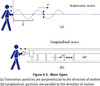14

## Longitudinal Waves

### sound waves particles of the wave oscillate along the direction of travel of the wave motion; that is, the wave particles are oscillating along the direction of energy transfer15

16

17

## Phase difference

### Destructive (vs constructive) interference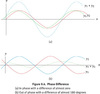18

## Traveling Wave19

20

21

22

23

24

25

26

27

28

## Sound Level (β )

### β = 10 log (I/Io) where Io is a reference intensity set at the threshold of hearing, 1 × 10− W/m2 decibels (dB) Calculate new sound level:βf = βi+ 10 log (If/Ii)where (If/Ii) is the ratio of the final intensity to the initial intensity.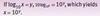29

30

31

32

33

## open pipes

### λ=(2L)/nwhere n is a positive nonzero integer (n = 1, 2, 3,… )Pattern: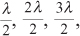etc.From relationship that f=v/λ, the possible frequencies are:f=(nv)/2Lwhere n is a positive nonzero integer (n = 1, 2, 3,… ).34

## harmonic series

### fundamental frequency( first harmonic): lowest frequency (longest wavelength) of a standing wave that can be supported in a given length of stringsecond harmonic: frequency of the standing wave given by n = 2 is known as the firstovertone or  This standing wave has one-half the wavelength and twice the frequency of the first harmonic. All the possible frequencies that the string can support form its harmonic series. The waveforms of the first three harmonics for a string of length L are shown.(Note: N stands for node and A stands for antinode.)35

## Closed Pipes

###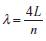λ=4L/nwhere n is odd integers only (n = 1, 3, 5,… ). The frequency of the standing wave in a closed pipe is f=nv/4L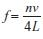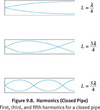36